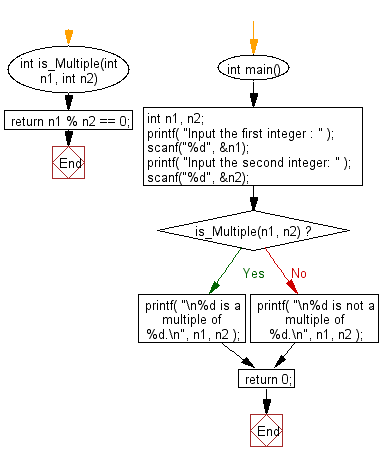﻿ C : 2 integers, check the first is a multiple of the second

# C Exercises: Reads in two integers and check whether the first integer is a multiple of the second integer

## C Basic Declarations and Expressions: Exercise-73 with Solution

Write a C program that reads two integers and checks whether the first integer is a multiple of the second integer.

Sample Input: 9 3

Sample Solution:

C Code:

``````#include<stdio.h>
// Function to check if n1 is a multiple of n2
int is_Multiple(int n1, int n2)
{
return n1 % n2 == 0;
}

int main()
{
int n1, n2;

// Prompt for user input
printf("Input the first integer : ");
scanf("%d", &n1);
printf("Input the second integer: ");
scanf("%d", &n2);

// Check if n1 is a multiple of n2 and print result
if(is_Multiple(n1, n2))
printf("\n%d is a multiple of %d.\n", n1, n2);
else
printf("\n%d is not a multiple of %d.\n", n1, n2);

return 0;
}
``````

Sample Output:

```Input the first integer : Input the second integer:
9 is a multiple of 3.
```

Pictorial Presentation:Flowchart:C programming Code Editor:

What is the difficulty level of this exercise?

Test your Programming skills with w3resource's quiz.

﻿